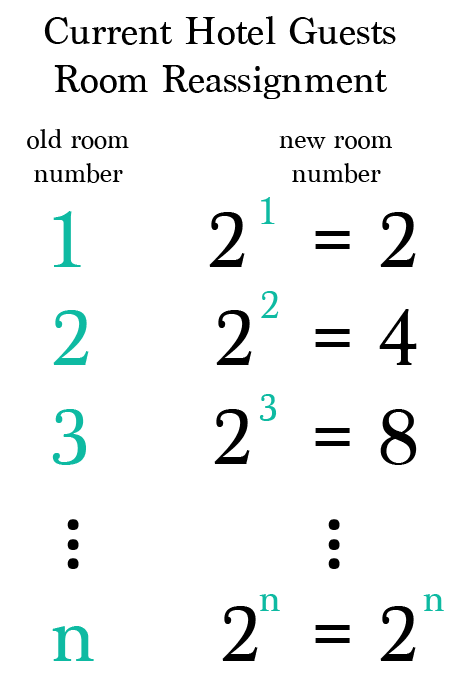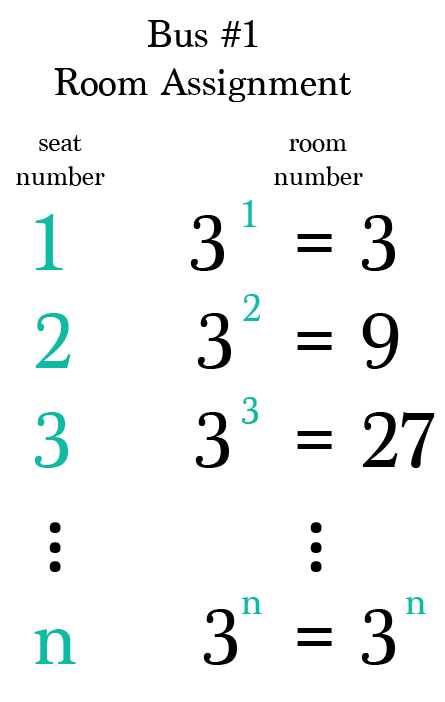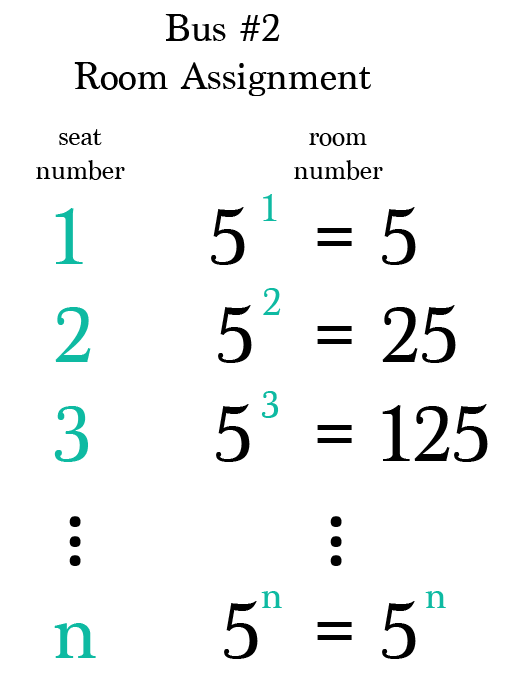Mathematics

# Countable Sets

474 views

 1 What are Countable Sets? 2 Hilbert’s Paradox 3 Further Examples 4 FAQs

## What are Countable Sets?

Countable sets are those sets that have their cardinality the same as that of a subset of Natural Numbers.

A countable set can either be a finite set as we can count the number of elements in a finite set or countably infinite set which we will learn further ahead.

A countable set is either a finite set or a countably infinite set. Whether finite or infinite, the elements of a countable set can always be counted one at a time and—although the counting may never finish—every element of the set is associated with a unique natural number.

Georg Cantor introduced the term countable set, contrasting sets that are countable with those that are uncountable.

Today, countable sets form the foundation of a important branch of mathematics called discrete mathematics. Discrete Mathematics has its use in almost all forms of applicative mathematics including and not limiting to almost all Technological Mathematics.

Countable and uncountable terms have often posed as the most confusing terms and have also led to many paradoxes. We will try to understand a famous one: Hilbert’s Paradox of the Grand Hotel.

Hilbert's paradox of the Grand Hotel is a mathematical paradox named after the German mathematician David Hilbert. Hilbert used it as an example to show how infinity does not act in the same way as regular numbers do.

Normal hotels have a set number of rooms. This number is finite. Once every room has been assigned to a guest, any new guest that wants a room and does not have one yet cannot be served - in other words, the hotel is fully booked.

Now suppose that there is a hotel that has an infinite number of rooms. As a convenience, the rooms have numbers, the first room has the number 1, the second has number 2, and so on.

If all the rooms are filled, it might appear that no more guests can be taken in, as in a hotel with a finite number of rooms. This is wrong, though. A room can be provided for another guest.

This can be done by moving the guest in room 1 to room 2, the guest in room 2 to room 3, and so on. In the general case, the guest in room $$n$$ will be moved to room $$n+1.$$

After all guests have moved, room 1 is empty, and the new guest now has room to occupy.

This shows how we can find a room for a new guest even if the hotel is already full, something that could not happen in any hotel with a finite number of rooms.

### Countable Sets-PDF

Countable sets are those sets that have their cardinality the same as that of a subset of Natural Numbers. Here is a downloadable PDF to explore more.

Finitely many new guests

Suppose a new guest arrives and wishes to be accommodated in the hotel. We can (simultaneously) move the guest currently in room 1 to room 2, the guest currently in room 2 to room 3, and so on, moving every guest from his current room $$n$$ to room $$n+1.$$ After this, room 1 is empty and the new guest can be moved into that room.

By repeating this procedure, it is possible to make room for any finite number of new guests.

### Infinitely many new guests

It is also possible to accommodate a countably infinite number of new guests: just move the person occupying room 1 to room 2, the guest occupying room 2 to room 4, and, in general, the guest occupying room $$n$$ to room $$2n$$ (2 times $$n$$), and all the odd-numbered rooms (which are countably infinite) will be free for the new guests.

### Infinitely many buses with infinitely many guests each

For starters, we’ll move the infinite set of guests currently in the hotel from their current room numbers (i.e. the natural numbers) to powers of the first prime number, 2.

So the guest in room 1 goes to room $$2^¹= 2,$$ the guest in room 2 goes to room $$2^² = 4,$$ and so on.Bus #1’s Room Assignments

Then we’ll take the guests from the first bus and assign them to powers of the next prime number, which is 3, using their seat numbers as the powers.

For example, in this first bus, we’ll move the guest from seat 1 to $$3^¹ = 3,$$ the guest from seat 2 to $$3^² = 9,$$ and so on.Bus #2’s Room Assignments

We’ll continue this pattern using the next prime number as the base for the next bus.And So On…

Because we have an infinite set of prime numbers, theoretically we can continue using the next prime as the base for each of the countably infinite busses. And since raising the prime number to a new power produces a brand new room number, we can locate rooms for the infinite guests on each bus.

Further Links of blogs and videos that one should refer for better understanding of Infinity and this paradox:

## Further Examples of Countable Sets:

Given that the concept of countability and cardinality is bit complex and not easy to understand in one go, we will try to explain it by taking various examples:

1. Whole Numbers (https://www.cuemath.com/learn/concepts/whole-numbers/)

2. Integers (https://www.cuemath.com/numbers/integers/)

## What is a countable set?

A countable set is a set of numbers that can have a one to one mapping with the set of natural numbers i.e. are either finite or countably infinite.

## What is an countable set?

An uncountable set is a set of numbers that don’t have a one to one mapping with the set of natural numbers i.e. they consists of infinite numbers.

## What are a few known examples of a countable set?

Examples of countable set include:

1. Natural Numbers
2. Even Numbers
3. Odd Numbers
4. Whole Numbers
5. Integers
6. Positive Integers
7. Negative Integers, etc.

## What are a few known examples of an uncountable set?

Examples of uncountable set include:

1. Rational Numbers
2. Irrational Numbers
3. Real Numbers
4. Complex Numbers
5. Imaginary Numbers, etc.

Award-winning math curriculum, FREE for a year